# 【数学建模学习笔记【集训十天】之第二天】

### 学习目录

• Numpy学习
• 1 Numpy 介绍与应用
• 1-1Numpy是什么：
• 1-2 为什么选择Numpy
• 1-2-1 代码更简洁
• 1-2-2 性能更高效
• 相关学习、代码如下：
• 线性图运行效果如下：
• 柱状图运行效果如下：
• 2 NumPy Ndarray 对象
• 相关学习、代码如下：
• 3 Numpy 数据类型：
• 相关学习、代码如下：
• 4 Numpy 数组属性
• 相关代码学习、如下
• Pandas学习
• 文件准备
• 1 pandas新增数据列
• 2 Pandas数据统计函数
• 3 Pandas对缺失值的处理
• 特殊Excel的读取、清洗、处理
• 遇到的问题：
• 总结：
• 每日一言：

• # Numpy学习

## 1 Numpy 介绍与应用

### 1-1Numpy是什么：

NumPy 是一个运行速度非常快的数学库，一个开源的的python科学计算库，主要用于数组、矩阵计算，包含：

• 一个强大的N维数组对象 ndarray
• 广播功能函数
• 整合 C/C++/Fortran 代码的工具
• 线性代数、傅里叶变换、随机数生成等功能
• ### 1-2 为什么选择Numpy

对于同样的数值计算任务，使用Numpy比直接编写原生python代码的优点有：

#### 1-2-1 代码更简洁

Numpy直接以数组、矩阵为粒度计算并且支撑大量的数学函数，而Python需要用for循环从底层实现

#### 1-2-2 性能更高效

Numpy的数组存储效率和输入输出计算性能，比Python使用List或者嵌套List好很多

`注意`：Numpy的数据存储和Python原生的List是不一样的
加上Numpy的大部分代码都是C语言实现的，这是Numpy比纯Python代码高效的原因

## 相关学习、代码如下：

`须提前安装好Numpy、pandas和matplotlib`

Numpy终端安装命令：pip install numpy
Pandas终端安装命令：pip install pandas
Matplotlib终端安装过命令：pip install matplotlib

像这样的【这个是Pycharm，用过的人都说好，啊哈哈】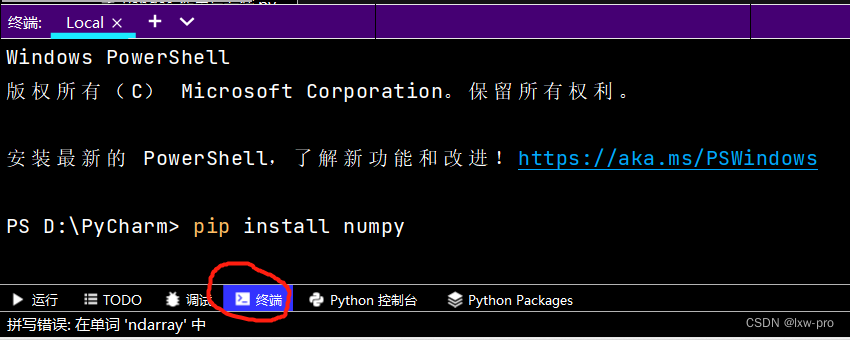``````# -*- coding = utf-8 -*-
# @Time : 2022/6/28 8:52
# @Author : lxw_pro
# @File : Numpy-介绍与应用-1.py
# @Software : PyCharm

# Numpy是Python各种数据科学类库的基础库
# 比如：Pandas，Scipy，Scikit_Learn等

# Numpy应用：
'''
NumPy 通常与 SciPy（Scientific Python）和 Matplotlib（绘图库）一起使用， 这种组合广泛用于替代 MatLab，是一个强大的科学计算环境，有助于我们通过 Python 学习数据科学或者机器学习。

SciPy 是一个开源的 Python 算法库和数学工具包。

SciPy 包含的模块有最优化、线性代数、积分、插值、特殊函数、快速傅里叶变换、信号处理和图像处理、常微分方程求解和其他科学与工程中常用的计算。

Matplotlib 是 Python 编程语言及其数值数学扩展包 NumPy 的可视化操作界面。它为利用通用的图形用户界面工具包，如 Tkinter, wxPython, Qt 或 GTK+ 向应用程序嵌入式绘图提供了应用程序接口（API）。

'''

# 安装 NumPy 最简单的方法就是使用 pip 工具：

# pip3 install --user numpy scipy matplotlib
# --user 选项可以设置只安装在当前的用户下，而不是写入到系统目录。

# 默认情况使用国外线路，国外太慢，我们使用清华的镜像就可以:
# pip install numpy scipy matplotlib -i.csv https://pypi.tuna.tsinghua.edu.cn/simple

# 这种pip安装是一种最简单、最轻量级的方法，当然，这里的前提是有Python包管理器

# 如若不行，可以安装Anaconda【目前应用较广泛】，这是一个开源的Python发行版
# 安装Anaconda地址：https://www.anaconda.com/

# 安装验证
# 测试是否安装成功
from numpy import *     # 导入 numpy 库

print(eye(4))           # 生成对角矩阵

# 查看版本：
import numpy as np

print(np.__version__)

# 实现2个数组的加法：

# 1-原生Python实现
def Py_sum(n):
a = [i**2 for i in range(n)]
b = [i**3 for i in range(n)]
# 创建一个空列表，便于后续存储
ab_sum = []
for i in range(n):
# 将a、b中对应的元素相加
ab_sum.append(a[i]+b[i])
return ab_sum

# 调用实现函数
print(Py_sum(10))

# 2-Numpy实现：
def np_sum(n):
c = np.arange(n) ** 2
d = np.arange(n) ** 3
return c+d

print(np_sum(10))

# 易看出使用Numpy代码简洁且运行效率快

# 测试1000，10W，以及100W的运行时间
# 做绘图对比：
import pandas as pd
# 输入数据
py_times = [1.72*1000, 202*1000, 1.92*1000]
np_times = [18.8, 14.9*1000, 17.8*10000]

# 创建Pandas的DataFrame类型数据
ch_lxw = pd.DataFrame({
'py_times': py_times,
'np_times': np_times    # 可加逗号
})

print(ch_lxw)

``````
``````import matplotlib.pyplot as plt

# 线性图
print(ch_lxw.plot())

# 柱状图
print(ch_lxw.plot.bar())

# 简易箱线图
print(ch_lxw.boxplot)

plt.show()
``````

#### 线性图运行效果如下：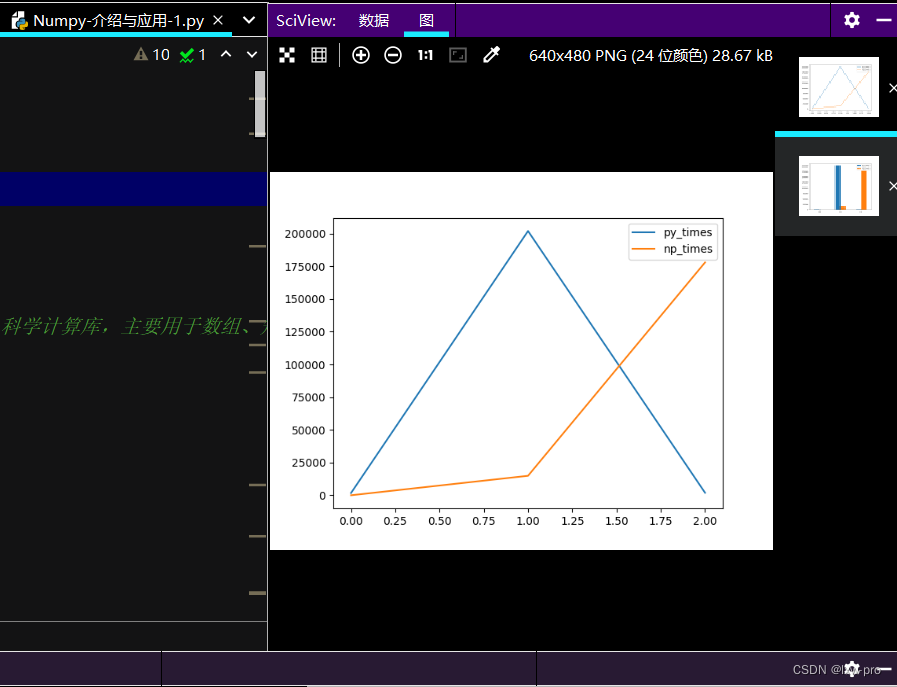#### 柱状图运行效果如下：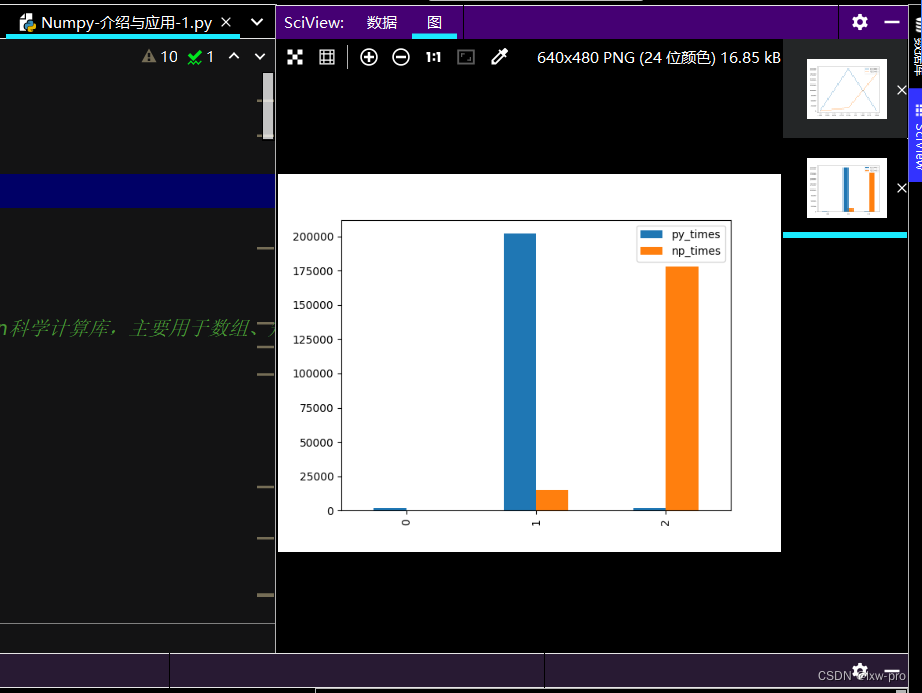## 2 NumPy Ndarray 对象

NumPy 最重要的一个特点是其 N 维数组对象 ndarray，它是一系列同类型数据的集合，以 0 下标为开始进行集合中元素的索引。

• ndarray 对象是用于存放同类型元素的多维数组，其中的每个元素在内存中都有相同存储大小的区域。
• ndarray 对象采用了数组的索引机制，将数组中的每个元素映射到内存块上，并且按照一定的布局对内存块进行排序（行或列）
• ndarray 内部由以下内容组成：

• 一个指向数据（内存或内存映射文件中的一块数据）的指针；

• 数据类型或 dtype，描述在数组中的固定大小值的格子；

• 一个表示数组形状（shape）的元组，表示各维度大小的元组；

• 一个跨度元组（stride），其中的整数指的是为了前进到当前维度下一个元素需要"跨过"的字节数。

• ## 相关学习、代码如下：

``````'''
创建一个 ndarray 只需调用 NumPy 的 array 函数即可：
numpy.array(object, dtype = None, copy = True, order = None, subok = False, ndmin = 0)

参数说明：

名称	描述
object	表示数组或嵌套的数列
dtype	表示数组元素的数据类型，可选
copy	表示对象是否需要复制，可选
order	创建数组的样式，C为行方向，F为列方向，A为任意方向（默认）
subok	默认返回一个与基类类型一致的数组
ndmin	指定生成数组的最小维度

'''

# ndarray 对象由计算机内存的连续一维部分组成，并结合索引模式，将每个元素映射到内存块中的一个位置。
# 内存块以行顺序(C样式)或列顺序(FORTRAN或MatLab风格，即前述的F样式)来保存元素

# 学好Numpy，便于后期对Pandas的数据处理

# 1:一维
import numpy as np

lxw = np.array([5, 2, 0])
print(lxw)

print()
# 2： 多于一个维度
import numpy as np

lxw2 = np.array([[1, 5, 9], [5, 2, 0]])
print(lxw2)

print()
# 3: 最小维度
import numpy as np

lxw3 = np.array([5, 2, 0, 1, 3, 1, 4], ndmin=2)     # ndmin: 指定生成数组的最小维度
print(lxw3)

print()
# 4： dtype参数
import numpy as np

lxw4 = np.array([3, 3, 4, 4], dtype=complex)        # dtype: 数组元素的数据类型[complex 复数】
print(lxw4)

``````

## 3 Numpy 数据类型：

numpy 支持的数据类型比 Python 内置的类型要多很多，基本上可以和 C 语言的数据类型对应上，其中部分类型对应为 Python 内置的类型.

常用 NumPy 基本类型

名称 描述
bool_ :【布尔型数据类型（True 或者 False）】
int_ : 【默认的整数类型（类似于 C 语言中的 long，int32 或 int64）】
intc :【与 C 的 int 类型一样，一般是 int32 或 int 64】
intp :【用于索引的整数类型（类似于 C 的 ssize_t，一般情况下仍然是 int32 或 int64）】
int8 :【字节（-128 to 127）】
int16 :【整数（-32768 to 32767）】
int32 :【整数（-2147483648 to 2147483647）】
int64 ：【整数（-9223372036854775808 to 9223372036854775807）】
uint8 ：【无符号整数（0 to 255）】
uint16 ：【无符号整数（0 to 65535）】
uint32 ：【无符号整数（0 to 4294967295）】
uint64 ：【无符号整数（0 to 18446744073709551615）】
float_ float64 ：【类型的简写】
float16 ：【半精度浮点数，包括：1 个符号位，5 个指数位，10 个尾数位】
float32 ：【单精度浮点数，包括：1 个符号位，8 个指数位，23 个尾数位】
float64 ：【双精度浮点数，包括：1 个符号位，11 个指数位，52 个尾数位】
complex_ complex128： 【类型的简写，即 128 位复数】
complex64 ：【复数，表示双 32 位浮点数（实数部分和虚数部分）】
complex128 ：【复数，表示双 64 位浮点数（实数部分和虚数部分）】

## 相关学习、代码如下：

``````
'''

# numpy 的数值类型实际上是 dtype 对象的实例，并对应唯一的字符，包括 np.bool_，np.int32，np.float32，等等。

'''

#　Numpy 类型对象：
'''
dtype 对象是使用以下语法构造的：

numpy.dtype(object, align, copy)

object - 要转换为的数据类型对象
align - 如果为 true，填充字段使其类似 C 的结构体。
copy - 复制 dtype 对象 ，如果为 false，则是对内置数据类型对象的引用

'''

# 1: 使用标量类型
import numpy as np

lxw = np.dtype(np.int32)
print(lxw)

print()
# 2: int8, int16, int32, int64 四种数据类型可以使用字符串 'i1', 'i2','i4','i8' 代替
import numpy as np

lxw2 = np.dtype('i8')       # int64
print(lxw2)

print()
# 3: 字节顺序标注
import numpy as np

lxw3 = np.dtype('<i4')      # int32
print(lxw3)

print()
# 4: 首先创建结构化数据类型
import numpy as np

lxw4 = np.dtype([('age', np.int8)])     # i1
print(lxw4)

print()
# 5: 将数据类型应用于 ndarray 对象
import numpy as np

lxw5 = np.dtype([('age', np.int32)])
a = np.array([(10,), (20,), (30,)], dtype=lxw5)
print(a)

print()
# 6: 类型字段名可以用于存取实际的 age 列
import numpy as np

lxw6 = np.dtype([('age', np.int64)])
a = np.array([(10,), (20,), (30,)], dtype=lxw6)
print(a['age'])

print()
# 7: 定义一个结构化数据类型 student，包含字符串字段 name，整数字段 age，及浮点字段 marks，并将这个 dtype 应用到 ndarray 对象
import numpy as np

student = np.dtype([('name', 'S20'), ('age', 'i2'), ('marks', 'f4')])
print(student)      # 运行结果：[('name', 'S20'), ('age', '<i2'), ('marks', '<f4')]

print()
# 8:
import numpy as np

student2 = np.dtype([('name','S20'), ('age', 'i1'), ('marks', 'f4')])
lxw = np.array([('lxw', 21, 52), ('cw', 22, 58)], dtype=student2)
print(lxw)          # 运行结果：[(b'lxw', 21, 52.) (b'cw', 22, 58.)]

# 每个内建类型都有一个唯一定义它的字符代码，如下：
'''
字符	对应类型
b	布尔型
i.csv	(有符号) 整型
u	无符号整型 integer
f	浮点型
c	复数浮点型
m	timedelta（时间间隔）
M	datetime（日期时间）
O	(Python) 对象
S, a	(byte-)字符串
U	Unicode
V	原始数据 (void)

'''

``````

## 4 Numpy 数组属性

在 NumPy中，每一个线性的数组称为是一个轴（axis），也就是维度（dimensions）。

比如说，二维数组相当于是两个一维数组，其中第一个一维数组中每个元素又是一个一维数组。

## 相关代码学习、如下

``````# -*- coding = utf-8 -*-
# @Time : 2022/6/28 16:52
# @Author : lxw_pro
# @File : Numpy-数组属性-4.py
# @Software : PyCharm

# NumPy 的数组中比较重要 ndarray 对象属性有：
'''
属性	            说明
ndarray.ndim	秩，即轴的数量或维度的数量
ndarray.shape	数组的维度，对于矩阵，n 行 m 列
ndarray.size	数组元素的总个数，相当于 .shape 中 n*m 的值
ndarray.dtype	ndarray 对象的元素类型
ndarray.itemsize	ndarray 对象中每个元素的大小，以字节为单位
ndarray.flags	ndarray 对象的内存信息
ndarray.real	ndarray元素的实部
ndarray.imag	ndarray 元素的虚部
ndarray.data	包含实际数组元素的缓冲区，由于一般通过数组的索引获取元素，所以通常不需要使用这个属性。

'''

# ndarray.ndim

# ndarray.ndim 用于返回数组的维数，等于秩。
import numpy as np

lxw = np.arange(36)
print(lxw.ndim)             # a 现只有一个维度

# 现调整其大小
a = lxw.reshape(2, 6, 3)    # 现在拥有三个维度
print(a.ndim)

print()
# ndarray.shape
# ndarray.shape 表示数组的维度，返回一个元组，这个元组的长度就是维度的数目，即 ndim 属性(秩)。比如，一个二维数组，其维度表示"行数"和"列数"。

# ndarray.shape 也可以用于调整数组大小。

import numpy as np

lxw2 = np.array([[169, 175, 165], [52, 55, 50]])
print(lxw2.shape)   # shape: 数组的维度

print()
# 调整数组大小:
import numpy as np

lxw3 = np.array([[123, 234, 345], [456, 567, 789]])
lxw3.shape = (3, 2)
print(lxw3)

print()
# NumPy 也提供了 reshape 函数来调整数组大小:
import numpy as np

lxw4 = np.array([[23, 543, 65], [32, 54, 76]])
c = lxw4.reshape(2, 3)  # reshape: 调整数组大小
print(c)

print()
# ndarray.itemsize
# ndarray.itemsize 以字节的形式返回数组中每一个元素的大小。

# 例如，一个元素类型为 float64 的数组 itemsize 属性值为 8(float64 占用 64 个 bits,
# 每个字节长度为 8，所以 64/8，占用 8 个字节），又如，一个元素类型为 complex32 的数组 item 属性为 4（32/8）

import numpy as np

# 数组的 dtype 为 int8（一个字节）
x = np.array([1, 2, 3, 4, 5], dtype=np.int8)
print(x.itemsize)

# 数组的dtypy现在为float64(八个字节）
y = np.array([1, 2, 3, 4, 5], dtype=np.float64)
print(y.itemsize)   # itemsize: 占用字节个数

# 拓展：
# 整体转化为整数型
print(np.array([3.5, 6.6, 8.9], dtype=int))

# 设置copy参数，默认为True
a = np.array([2, 5, 6, 8, 9])
b = np.array(a)                     # 复制a
print(b)                            # 控制台打印b
print(f'a: {id(a)}, b: {id(b)}')     # 可打印出a和b的内存地址

print('='*20)
# 类似于列表的引用赋值
b = a
print(f'a: {id(a)}, b: {id(b)}')

# 创建一个矩阵
lxw5 = np.mat([1, 2, 3, 4, 5])
print(type(lxw5))   # 矩阵类型： <class 'numpy.matrix'>

# 复制出副本，并保持原类型
yy = np.array(lxw5, subok=True)
print(type(yy))

# 只复制副本，不管其类型
by = np.array(lxw5, subok=False)    # False: 使用数组的数据类型
print(type(by))

print(id(yy), id(by))

print('='*20)
# 使用数组的copy()方法：
c = np.array([2, 5, 6, 2])
cp = c.copy()
print(id(c), id(cp))

print()
# ndarray.flags
'''
ndarray.flags 返回 ndarray 对象的内存信息，包含以下属性：

属性	描述
C_CONTIGUOUS (C)	数据是在一个单一的C风格的连续段中
F_CONTIGUOUS (F)	数据是在一个单一的Fortran风格的连续段中
OWNDATA (O)	数组拥有它所使用的内存或从另一个对象中借用它
WRITEABLE (W)	数据区域可以被写入，将该值设置为 False，则数据为只读
ALIGNED (A)	数据和所有元素都适当地对齐到硬件上
UPDATEIFCOPY (U)	这个数组是其它数组的一个副本，当这个数组被释放时，原数组的内容将被更新

'''

import numpy as np

lxw4 = np.array([1, 3, 5, 6, 7])
print(lxw4.flags)   # flags: 其内存信息

``````

`学习来源于哔哩哔哩和菜鸟教程`
学习可自行点击：【菜鸟教程】
【2022B站最新最好的数据分析课程推荐】

# Pandas学习

当然，做这些的前提是首先把文件准备好

#### 文件准备

文件太长，故只截取了部分，当然，此文件可自行弄类似的也可以！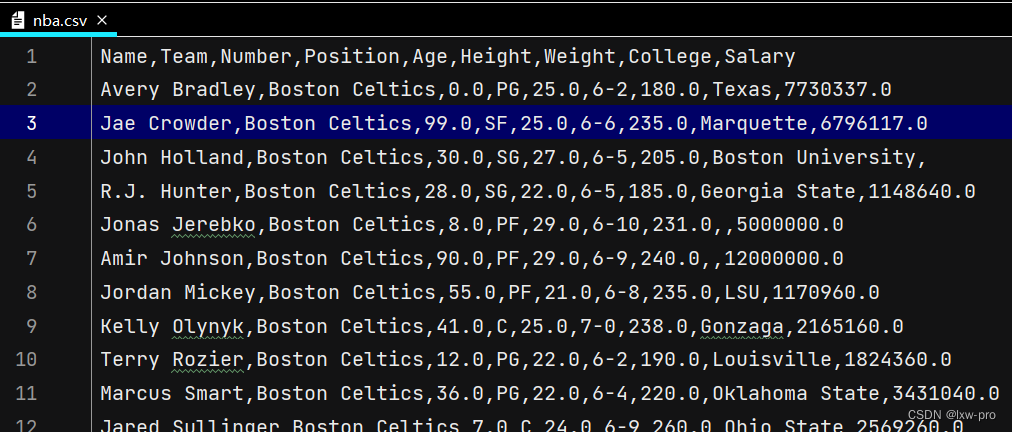## 1 pandas新增数据列

在进行数据分析时，经常需要按照一定条件创造新的数据列，然后再进一步分析

• 直接赋值
• df.apply()方法
• df.assign()方法
• 按条件进行分组分别赋值
• ``````# -*- coding = utf-8 -*-
# @Time : 2022/6/28 16:20
# @Author : lxw_pro
# @File : pandas新增数据列.py
# @Software : PyCharm

# 1:
import pandas as pd

# 读取数据

df = pd.DataFrame(lxw)

# print(df)

df['lrl'] = df['lrl'].map(lambda x: x.rstrip('%'))

# print(df)

df.loc[:, 'jf'] = df['yye'] - df['sku_cost_prc']

# 返回的是Series

# 2:
def get_cha(n):
if n['yye'] > 5:
return '高价'
elif n['yye'] < 2:
return '低价'
else:
return '正常价'

df.loc[:, 'yye_type'] = df.apply(get_cha, axis=1)

print(df['yye_type'].value_counts())

# 3:
# 可同时添加多个新列

print(df.assign(
yye_bh=lambda x: x['yye']*2-3,
sl_zj=lambda x: x['sku_cnt']*6

# 4:

# 按条件先选择数据，然后对这部分数据赋值新列

# 先创建空列
df['zyye_type'] = ''

df.loc[df['yye'] - df['sku_cnt']>8, 'zyye_type'] = '高'
df.loc[df['yye'] - df['sku_cnt'] <= 8, 'zyye_type'] = '低'

``````

下面分别是每个小问对应运行效果：

1：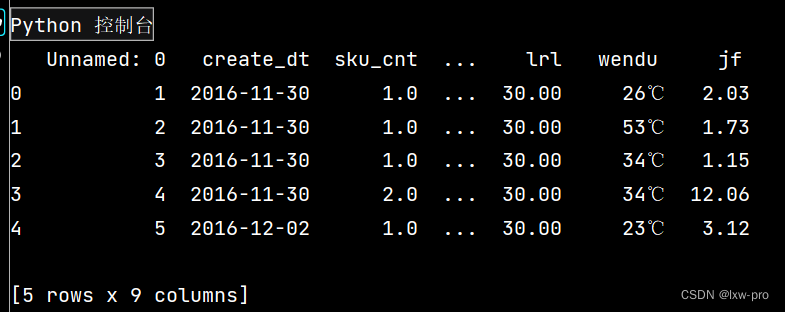2：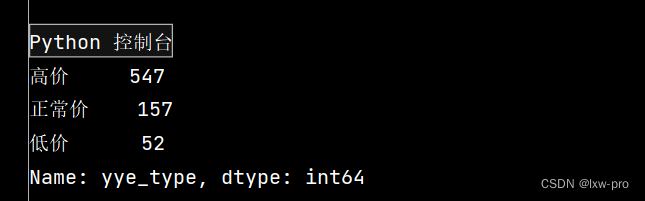3：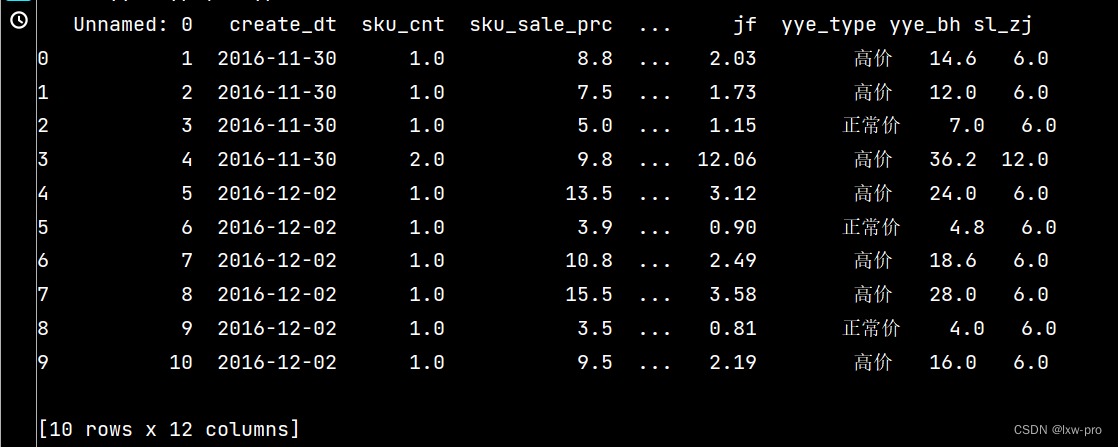4：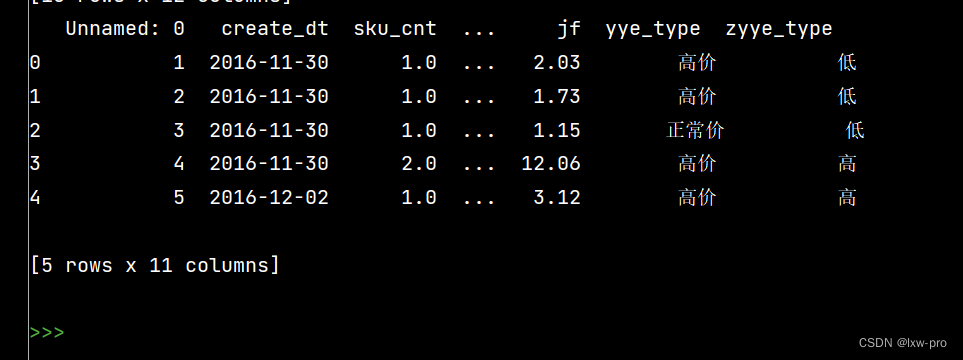## 2 Pandas数据统计函数

``````# Pandas数据统计函数

'''
1-汇总类统计
2-唯一去重和按值计数
3-相关系数和协方差

'''
import pandas as pd

# 1:

# 一下子提取所有数字列统计结果
print(lxw.describe())

# 查看单个Series的数据
print(lxw['Age'].mean())

# 年龄最大
print(lxw['Age'].max())

# 体重最轻
print(lxw['Weight'].min())

# 2:

# 2-1 唯一性去重【一般不用于数值项，而是枚举、分类项】
print(lxw['Height'].unique())

print(lxw['Team'].unique())

# 2-2 按值计算
print(lxw['Age'].value_counts())

print(lxw['Team'].value_counts())

# 3:

# 应用：股票涨跌、产品销量波动等等

'''
【来自知乎】
对于两个变量X、Y：
1-协方差：衡量同向程度程度，如果协方差为正，说明X、Y同向变化，协方差越大说明同向程度越高；
如果协方差为负，说明X、Y反向运动，协方差越小说明方向程度越高。
2-相关系数：衡量相似度程度，当他们的相关系数为1时，说明两个变量变化时的正向相似度最大，
当相关系数为-1，说明两个变化时的反向相似度最大。

'''

# 协方差矩阵：
print(lxw.cov())

# 相关系数矩阵：
print(lxw.corr())

# 单独查看年龄和体重的相关系数
print(lxw['Age'].corr(lxw['Weight']))

# Age和Salary的相关系数
print(lxw['Age'].corr(lxw['Salary']))

# 注意看括号内的相减
print(lxw['Age'].corr(lxw['Salary']-lxw['Weight']))

``````

1: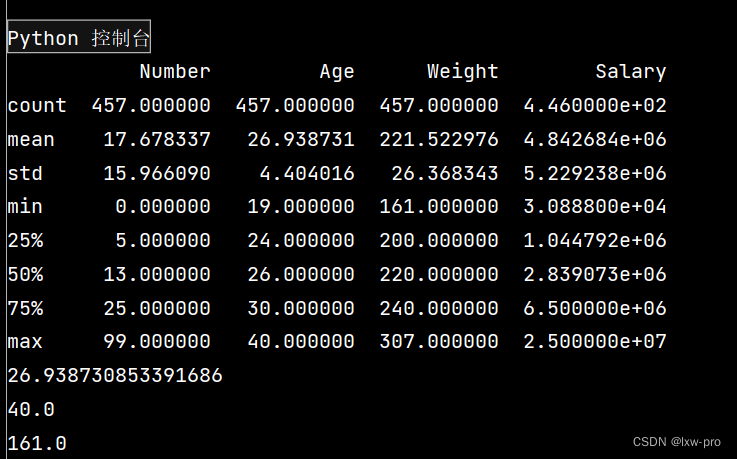2-1: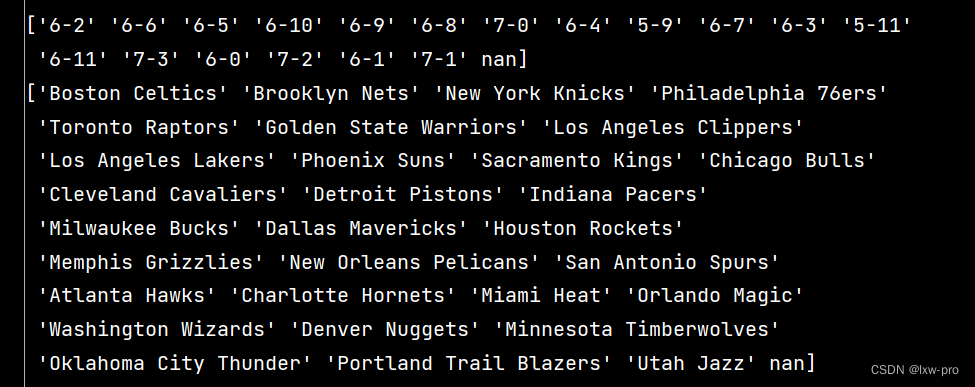部分2-2：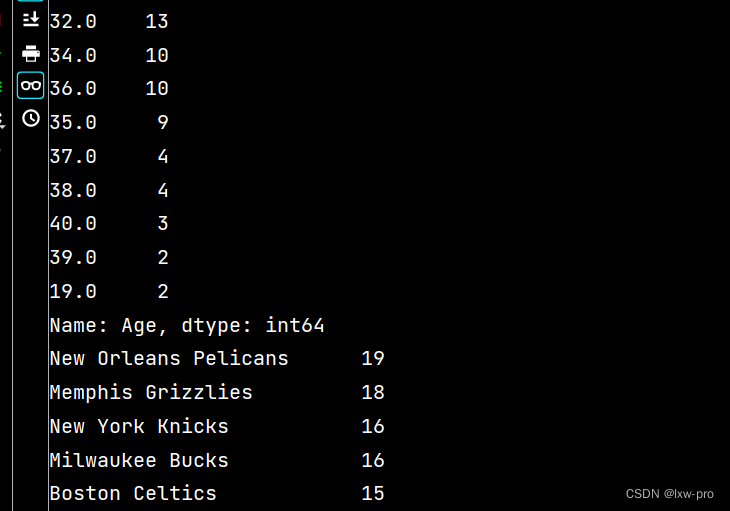3：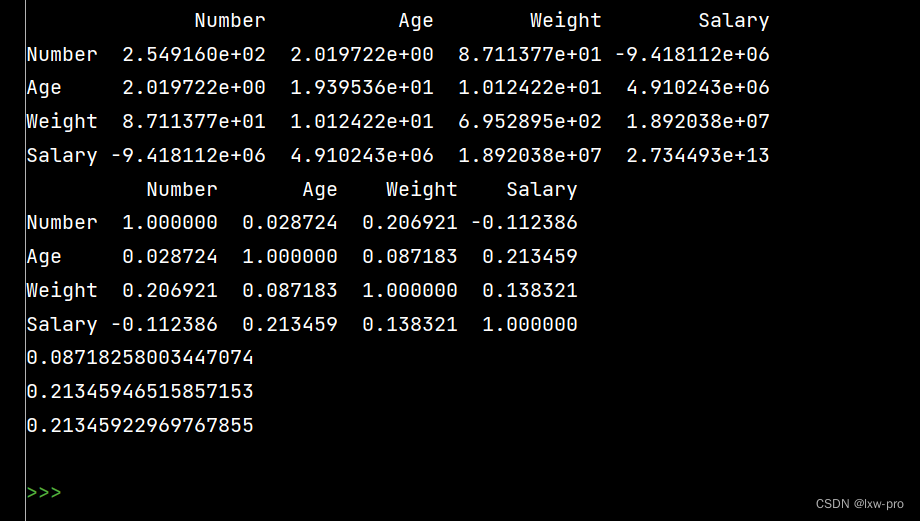## 3 Pandas对缺失值的处理

### 特殊Excel的读取、清洗、处理

``````# -*- coding = utf-8 -*-
# @Time : 2022/6/28 20:00
# @Author : lxw_pro
# @File : pandas缺失值处理-7.py
# @Software : PyCharm

# Pandas对缺失值的处理
'''
函数用法：
1-isnull和notnull: 检测是否有控制，可用于dataframe和series
2-dropna: 丢弃、删除缺失值
2-1 axis: 删除行还是列，{0 or 'index', 1 or 'columns'}, default()
2-2 how: 如果等于any， 则任何值都为空，都删除；如果等于all所有值都为空，才删除
2-3 inplace: 如果为True，则修改当前dataframe,否则返回新的dataframe
2-4 value: 用于填充的值，可以是单个值，或者字典（key是列名，value是值）
2-5 method: 等于ffill使用前一个不为空的值填充forword fill;等于bfill使用后一个不为空的值填充backword fill
2-6 axis: 按行还是按列填充，{0 or "index", 1 or "columns"}
2-7 inplace: 如果为True则修改当前dataframe，否则返回新的dataframe

'''

# 特殊Excel的读取、清洗、处理

import pandas as pd
# 1: 读取excel时，忽略前几个空行

stu = pd.read_excel("Score表.xlsx", skiprows=14)     # skiprows: 控制在几行以下

print(stu)

# 2: 检测空值
print(stu.isnull())

print(stu['成绩'].isnull())

print(stu['成绩'].notnull())

# 筛选没有空成绩的所有行
print(stu.loc[stu['成绩'].notnull(), :])

# 3: 删除全是空值的列：

# axis: 删除行还是列，{0 or 'index', 1 or 'columns'}, default()
# how: 如果等于any， 则任何值都为空，都删除；如果等于all所有值都为空，才删除
# inplace: 如果为True则修改当前dataframe，否则返回新的dataframe
stu.dropna(axis="columns",  how="all", inplace=True)

print(stu)

# 4: 删除全是空值的行：

stu.dropna(axis="index", how="all", inplace=True)

print(stu)

# 5: 将成绩列为空的填充为0分：
stu.fillna({"成绩": 0})

print(stu)

# 同上：
stu.loc[:, '成绩'] = stu['成绩'].fillna(0)

print(stu)

# 6: 将姓名的缺失值填充【使用前面的有效值填充，用ffill： forward fill】
stu.loc[:, '姓名'] = stu['姓名'].fillna(method='ffill')

print(stu)

# 7: 将清洗好的Excel保存:
stu.to_excel("Score成绩_clean.xlsx", index=False)

``````

1: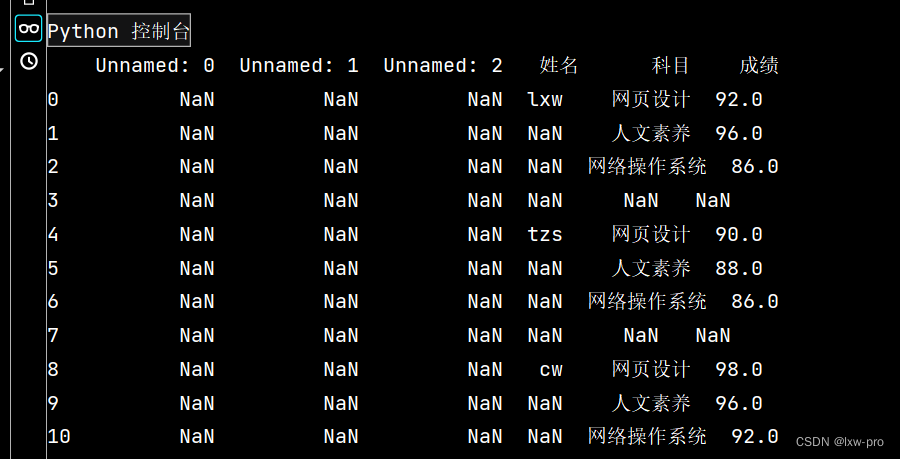2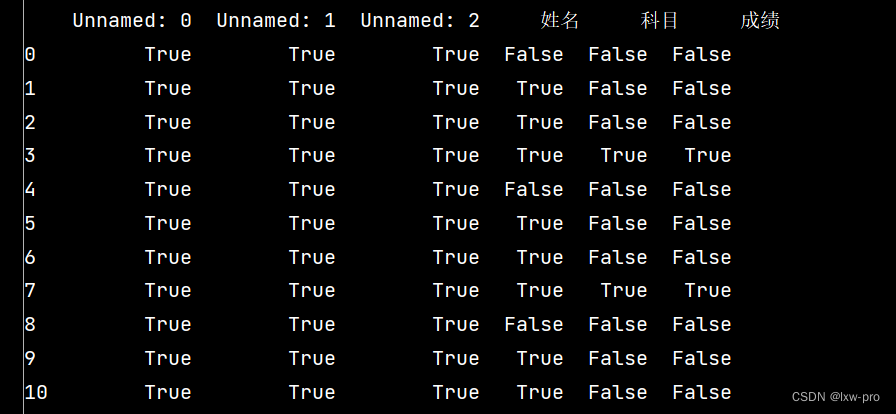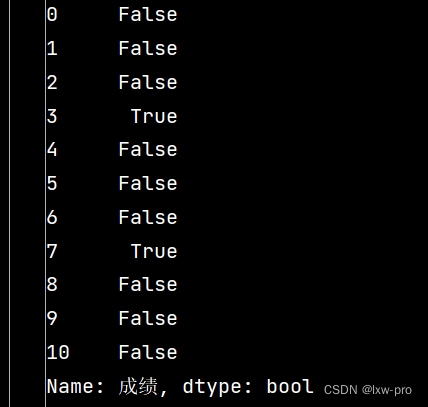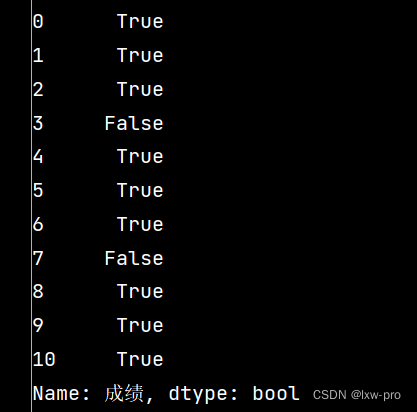3: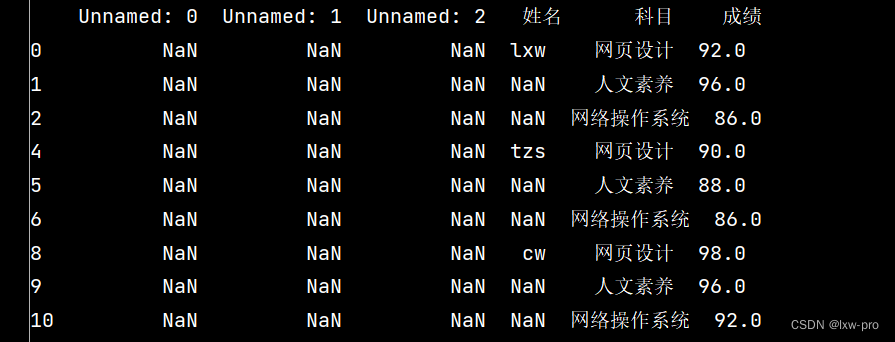4: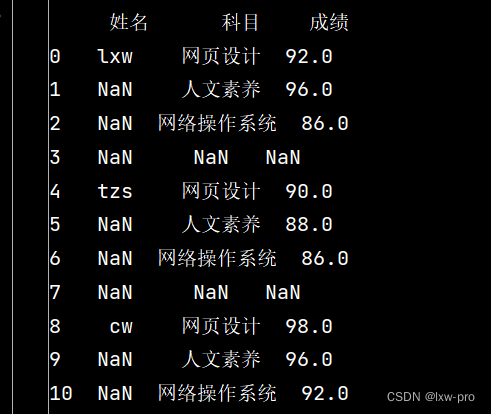5: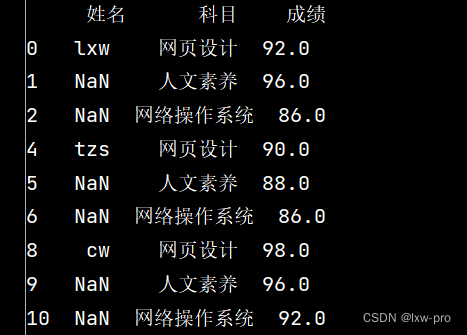6: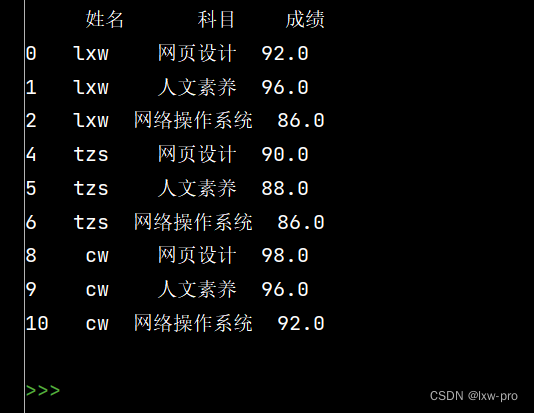上一章链接：【数学建模学习笔记【集训十天】之第一天】

`注`：模型和算法会在后面的文章陆续记写出来！！！

## 遇到的问题：

1-在学习Numpy时遇到线性图和柱状图时遇到 一些问题，后面上网查阅之后问题才得以解决。
2-学习Pandas当中遇到了类似字符串的处理出了一些问题，还是得以一顿午觉的协助破解难题。【因此，遇到难题时，要么跳开等会回来在开，要么去做其他事，待会再做，效果就会有异常的好】

### 总结：

今天我学习了处理python数据分析的另一个库——Numpy，刚开始接触这个库的时候真的感觉没什么意思，可学的越深入一点，越觉得越有意思，当然，昨天的那个库也挺不错的，主要是Numpy这个是学Pandas的基础，得打好基础，当然也不会落下Pandas的学习！

## 每日一言：

每天都是一个崭新的日子。走运固然不错，不过我宁可保持精确。那样，机会来临时，你已经做好了准备。

来源：lxw-pro